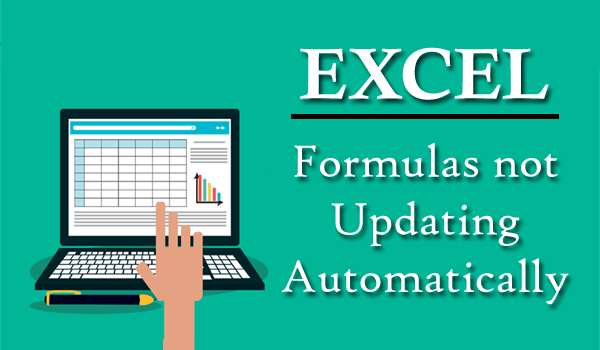Summary: This article provides complete information about how to fix Excel formulas not updating or calculating automatically and the reasons why the formulas are not updating automatically in Excel.

Microsoft Excel is one of the most useful programs in modern computing but what if Excel is without formulas or imaging using Microsoft Excel without formulas.

Well, I guess no one can, isn’t it…!!!

MS Excel is all about formulas and millions of people use the Excel spreadsheets for performing plenty of tasks like maintaining records to advanced data analysis using complex formulas.

So, unquestionably Excel formulas are very vital in Microsoft Excel. But there are cases when the Excel formulas stop working suddenly and trigger various problems related to formulas.

And Excel formulas do not update automatically or Excel formula not calculating is one of them. If you are the one struggling with the same issue then this tutorial is for you.

Just go through the article thoroughly and follow the given fixes one by one to make your Excel formulas updating automatically again.

### Why Are My Formulas not Automatically Updating in Excel?

Here check out the possible reasons for the Excel formulas not updating automatically issue:

1. Your calculation is configured to “Manual”
2. The show formulas button is turned on
3. The cell is formatted as Text
4. Before the Equal sign, you have entered space.

So these are the common reasons why the Excel formulas not updating automatically, now try the given fixes.

### Solution 1: Calculation is Set to “Manual”

This is one of the most common reasons responsible for triggering the Excel formulas do not update automatically.

So, check if the calculation option is configured to “manual” then set to “Automatic” to fix the problem.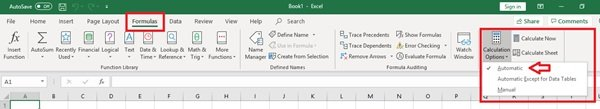Well, the steps may vary as per your Excel version so here follow the steps for the different Excel versions.

• Excel 2003 – Click Tools > Options > Calculation > Calculation > Automatic.
• In Excel 2007 – Click Office button > Excel options > Formulas > Workbook Calculation > Automatic.
• Excel 2010, 2013, & 2016 – Go to File > Options > Formulas > Calculation options section > under workbook calculation > choose Automatic.

Now check if the formulas not updating automatically issue is resolved or not.

### Solution 2: Show Formulas Button is Turned On

If the above-given solution won’t work for you then check if the Show Formulas button on the Formulas tab is turned on.

Then also there are chances the formulas do not work or update. Well, this button is mainly used when auditing formulas this displays formulas in place of the end result.

Though, this tab is helpful if fixing formula malfunctioning.

And to change it click Show Formulas button and Turn it Off.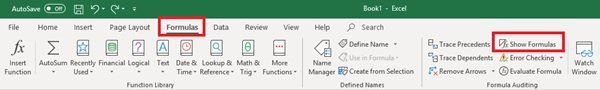Verify if the formulas not updating in Excel is resolved or not.

### Solution 3: Cell is formatted as Text

If you have accidentally formatted the cells including formulas as Text, then this is what causes the problem. As when the cell is in Text format, the cell will not calculate formulas automatically.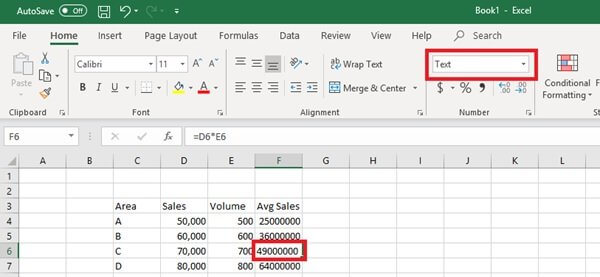So, click on the cell and of the Home, tab check the Number group > if it displays Text > then change it to General.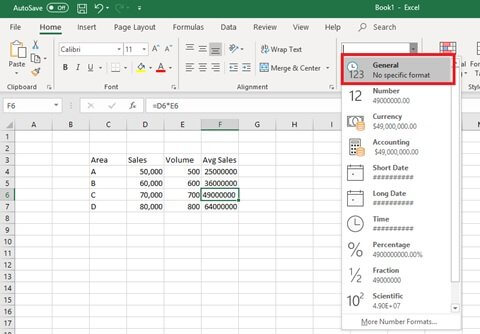And you can now recalculate the formula in the cell by double-click on the cell > press Enter.

### Solution 4:  Check for Circular References

Check circular reference as this can be another culprit that causes Excel formulas not updating.

The circular reference is a formula that includes itself in its calculation or refers to another cell that depends on itself.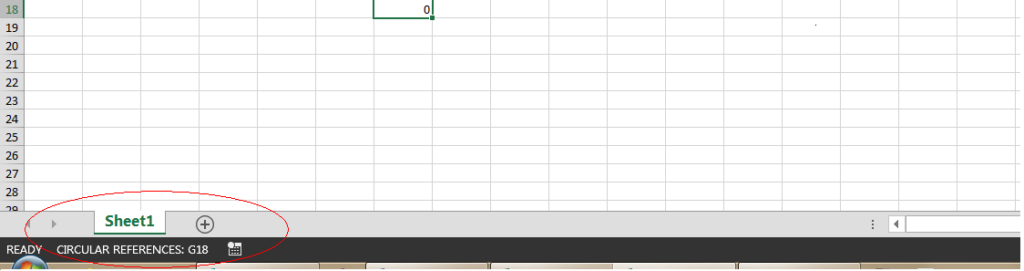And in some cases, this prevents the Excel from calculating the formula. So check it and correct the circular reference and recalculate your spreadsheet.

### Solution 5: Space Entered before the Equal Sign

This is the last solution that causes the Excel formulas not updating automatically.

Well, while typing formula if you by mistakenly enter a space before the “Equal (=)”, then the formula will not calculate.

This mistake is quite tricky and difficult to notice and left unidentified.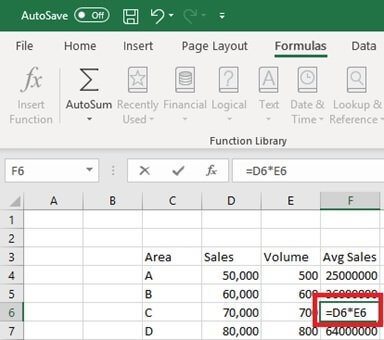Double click the cell and check if there is a space > delete it and the formula will update.

That’s it the given formulas tips will work for you and the issue is resolved now.

### Conclusion:

So, this is all about the formulas not updating automatically.

It is estimated that after following the aforementioned solutions you are able to fix formulas not automatically updating in Excel issue.

This is a common issue encountered by the regular Excel users once, so now you can tackle them easily.

However, if you are looking to become more advanced in Excel and learn tricks to make use of the Microsoft Excel advanced functions easily then visit this – Learn MS Excel

If, in case you have any additional questions, query, or fixes concerning the ones presented, then visit our Repair MS Excel Ask Question5 Easy Solutions to Fix Excel Formulas not Updating Automatically
SummaryArticle Name
5 Easy Solutions to Fix Excel Formulas not Updating Automatically
Description
Learn possible reasons why Excel formulas not updating automatically & try the fixes such as set calculation to automatic, check if formula button is turned ON, etc. to solve Excel formulas not working…
Author
Publisher Name
Repair MS Excel Blogs
Publisher Logo
• 1
Share